[中文] Search Internet Search HelloDBA
Oracle Technology Site. email: fuyuncat@gmail.com  MSN: fuyuncat@hotmail.com# Full Table Scan cost formula cracking(2)

[中文]

Author:  fuyuncat

Source:  www.HelloDBA.com

Date:  2009-08-01 15:03:36

Share to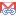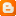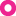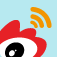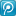## 3.1.System Statistic Data

First, I will look on the impact of system statistics data on the COST_CPU. These data include CPUSPEED, IOTFRSPEED, IOSEEKTIM, SREADTIM and MREADTIM. Here we adopt noworkload mode, SREADTIM, MREADTIM value is calculated from IOTFRSPEED & IOSEEKTIM and MBRC & BLKSIZ, so we only need derived MBRC, BLKSIZ in the formula, no need work on SREADTIM, MREADTIM. I will derive these two factors in system parameters part.

### 3.1.1.CPUSPEED

I will modify the CPUSEED value via BMS_STATS.set_system_stats, then compare the changes with the baseline (blue words)

 CPUSPEED Table Scan Cost_cpu 1000 2542429 2000 2542429 4000 2542429 8000 2542429

We can find the CPUSPEED will not impact the COST_CPU, here is the 1st rule we get

RULE1CPUSPEED has NO business with #CPUCYCLES.

### 3.1.2.IOTFRSPEED

After modify the IOTFRSPEED, we also find it not impact on COST_CPU.

 IOTFRSPEED Table Scan Cost_cpu 1024 2542429 2048 2542429 4096 2542429 8192 2542429

RULE 2IOTFRSPEED has no business with #CPUCYCLES.

### 3.1.3.IOSEEKTIM

After modify the IOSEEKTIM, we also find it not impact on COST_CPU.

 IOSEEKTIM Table Scan Cost_cpu 10 2542429 20 2542429 40 2542429 80 2542429

RULE 2IOSEEKTIM has no business with #CPUCYCLES.

## 3.2. Tables statistics data

Here is the table statistics data: data block number under HWM (we will call it data blocks, TABBLKS); the number of records in table, TABROWS and the average length of table rows, AVGLEN. Base on the same methods, we can found AVGLEN will not impact on COST_CPU.

### 3.2.1.TABROWS

We use dbms_stats.set_table_stats to modify TABROWS and compare the changes:

 TABROWS Table Scan Cost_cpu CPU_A CPU_B 10000 2542429 2400000 142429 20000 4942429 4800000 142429 40000 9742429 9600000 142429 80000 19342429 19200000 142429

We can see it changes is linear, then I draw curves:We can see that it is a slash, which means there is a proportional relationship between them in! We can solve the system of linear equations of two unknowns:

10000*X + Z = 2542429        … …   (1)

20000*X + Z = 4942429        … …   (2)

(2) – (1) =>

10000*X = 2400000

=>

X = 2400000 / 10000 = 240

=>

Z = 142429

Here we get the first formula:

Formula 1: COST_CPU = 240 * TABROWS + 142429

Among it:

CPU_A240 * TABROWS

CPU_B142429

Put 40000 and 80000 we not used in deducing process into the formula, the result is same as in trace file.
However,  240 &142429 should not be 2 simple constants, they must be subject to the remaining factors, we deduced how the other factors work in these 2 constants. According to formula one, in follow process, I will divide COST_CPU into two parts, tentatively called CPU_A, CPU_B (see above form).

### 3.2.2.TABBLKS

Continue work on TABBLKS

 TABBLKS Table Scan Cost_cpu CPU_A CPU_B 10 2471214 2400000 71214 20 2542429 2400000 142429 40 2684858 2400000 284858 80 2969715 2400000 569715

Basically, changes at here is also linear. There is some deviations occur, we assume it was caused by ROUNDING. We first divide baseline data (TABBLKS = 20) into A, B part, following this rule, continue divide the other data and found that CPU_B is linear. Here we get an assumptions 1: TABROWS will only affect on CPU_A part, not on CPU_B.

Solve the linear equations of one unknown (TABROWS here is 10000):

240 * 10000 + X * 10 = 2471214

=>

X = 7121.4

The second formula is obtained:

Formula 2: COST_CPU = 240 * TABROWS + 7121.4 * TABBLKS

Among it

CPU_A240 * TABROWS

CPU_B7121.4 * TABBLKS

As this formula is based on the assumption that before, in order to prove this assumption, I will try number of other TABBLKS into the equation, the results are in line, we deem the assumption is valid.

## 3.3. System parameters

There are some parameters will affect the cost computing. However, through the above-mentioned method, I found that the most of parameters does not affect the COST_CPU in Full Table Scan, including _cpu_to_io, _db_file_optimizer_read_count and so on. The only effective parameter is _optimizer_block_size.

### 3.3.1._optimizer_block_size

_optimizer_block_size (we name it as BLKSIZ) is the data block size that optimizer use to calculate the cost. Through IQ method, I took much of effort to find its position in the formula. Let us look at the COST_CPU changes when modify it (here we changed the base table, TABROWS = 1000000, TABBLKS = 1000, data is different from before, but it does not affect the derivation):

 _optimizer_block_size Table Scan Final Cost_CPU 4096 245810720 8192 247121440 16384 249742880

First, we can also find it’s linear:

COST_CPU = X*BLKSIZ + Z

And we try to divide the data into 2 parts as before:

 _optimizer_block_size Table Scan Final Cost_CPU CPU_A CPU_B 4096 245810720 240000000 5810720 8192 247121440 240000000 7121440 16384 249742880 240000000 9742880

This decomposition is based on the assumption (assumption 2) that CPU_A part was not affected by BLKSIZ. From the results, CPU_B changing is linear, also can be deduced. The draft equation is:

COST_CPU = CPU_A + X*BLKSIZ + Z

Base on formula 2, convert it:

COST_CPU = 240*TABROWS + TABBLKS*(X*TABSIZ + Z)

And solve the system of linear equations of two unknowns:

240*1000000 + 1000*(X*4096 + Z) = 245810720      … …      (1)

240*1000000 + 1000*(X*8192 + Z) = 247121440      … …      (2)

(2) – (1) =>

1000*4096*X = 1310720

=>

X = 0.32

=>

Z = 4500

We got the new formula:

Formula 3COST_CPU = 240*TABROWS + TABBLKS*( 0.32*TABSIZ + 4500 )

= 240*TABROWS + 0.32*TABBLKS*TABSIZ + 4500*TABBLKS

Among it

CPU_A240 * TABROWS

CPU_B0.32*TABBLKS*TABSIZ + 4500*TABBLKS

I also used some random data input into the formula, found all of them passed.

Note: when the block size of the tablespace on which the table located, the BLKSIZ will be the block size of the tablespace.

## 3.4. The query

This is the most complex part. Some of these factors are not expected, they are found from the special cases, and finally confirmed by assumption & testing.

### 3.4.1.Query columns number

This factor was not taken into account initially, however, after I traced a real database table, found the formula 3 can not calculate the correct result. It tells me there must be some other factors affected the results. By comparing the real table and baseline table, first I found is that column number of the table is different. So I try to increase the number of columns of the base table, and then compared with the data:

TABROWS: 1000000; TABBLKS: 1000

 COLNUMS COST_CPU CPU_B CPU_A 5 237121440 7121440 230000000 6 257121440 7121440 250000000 7 277121440 7121440 270000000 8 297121440 7121440 290000000

First of all, I decompose the data base on the formula 3. From the result, we can easily assume that number of columns only affect part of CPU_A (assumption 3).

The change is linear. we can get the system of linear equations of two unknowns:

1000000*(X*5 + Z) = 230000000      … …      (1)

1000000*(X*6 + Z) = 250000000      … …      (2)

(2)/1000000 – (1)/1000000 =>

X = 20

=>

Z = 130

We got the new formula:

COST_CPU = (20*COLUMNS + 130)*TABROWS + 0.32*TABBLKS*TABSIZ + 4500*TABBLKS

And it can be proved by the random data.

But, wait, is the number of columns the really factor in this formula? Look at the statement we did the trace: "SELECT * FROM TABLE". Please note here is * we used, which illustrate that there is another number is equal to COLNUMS in this case: the number of query columns. In order to identify who is really working or both of them are the factors, I have 2 sets of testing data.
The first set is the different number of query columns with the same the number of table columns:

 QRYCOLNUMS COST_CPU CPU_B cpu_A 2 208121440 7121440 201000000 3 209121440 7121440 202000000 4 210121440 7121440 203000000 5 211121440 7121440 204000000

The second set is the different number of table columns with the same the number of query columns:

 COLNUMS COST_CPU CPU_B cpu_A 5 208121440 7121440 201000000 6 208121440 7121440 201000000 7 208121440 7121440 201000000 8 208121440 7121440 201000000

From the result, we can found it’s the query columns number actually affected the COST_CPU, instead of the table columns number. Then the new formula is:

Formula 4: COST_CPU = (20*QRYCOLUMNS + 130)*TABROWS + 0.32*TABBLKS*TABSIZ + 4500*TABBLKS

Among it:

CPU_A(20*QRYCOLUMNS + 130) * TABROWS

CPU_B0.32*TABBLKS*TABSIZ + 4500*TABBLKS

P.S. During the testing, I also found putting function one the query column or involving in constant will not affect the result, that is, below the 3 statements is the same cost:

select a, b from T_PEEKING11 a;

select a, to_number(b) from T_PEEKING11 a;

select a, 6, 1 from T_PEEKING11 a;

In the following derivation process on other factors, I got assumption 4: they all just affect the CPU_A. Based on this assumption, in order to simplify the derivation process, I will spin off CPU_A part: CPU_A = TYPFAC * TABROWS, and what we need to do is to derive the TYPFAC. In this step, the TYPFAC formula is:

Formula 5TYPFAC = CPU_A/TABROWS

Formula 6TYPFAC = 20*QRYCOLNUMS + 130

So far, we have discovered the cost formula of Full Table Scan in the case of "SELECT COLA, ..., COLN FROM TABLE". At least, in my tracing on the real table of production, did not find other any factor.

### 3.4.2.Filter

Note: To simplify the derivation process, what we used here are bind variables.

From the process that a statement is proceed in Oracle, we know that the Row Source Generator will process the result set, and therefore the size of the final result set must become one of the factors that affect the cost. We can easily see the impact by comparing the data:

 Filter COST_CPU CPU_B cpu_A None 237121440 7121440 204000000 A < :V1 211121440 7121440 201000000

We can see the change. However, what are the real factors, and how can I derive the equation? We can foresee many related factors, including the statistical data of column, the number of columns in filter, filter condition, column data types, and data length. As a result of can not be determined these factors, there are many mistakes during my deriving process like we ever misunderstand the number of table columns as query column number, and it take much of effort. In order to make it clear, I will not reproduce the mistakes in this article.

### 3.4.3.The column statistics data

First of all I can think of is that the column statistics data, including the average length of the column (AvgLen), the distinct value number of the column (NDV), the value of the number of NULL (Nulls), column density. we are able to modify these data through dbms_stats.set_column_stats, data to prove that the information did not affect the COST_CPU.